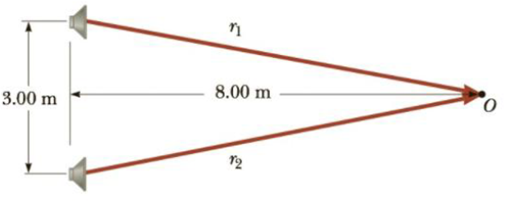Chapter 14, Problem 40P

Chapter
Section
Textbook Problem

Two loudspeakers are placed above and below each other, as in Figure P14.40 and driven by the same source at a frequency of 4.50 × 102 Hz. An observer is in front of the speakers (to the right) at point O, at the same distance from each speaker. What minimum vertical distance upward should the top speaker be moved to create destructive interference at point O?Figure P14.40

To determine
The minimum vertical distance upward should the top speaker be moved to create destructive interference at point O.

Explanation

Given Info: Frequency of sound from the speaker is 4.50×102Hz .

Formula to calculate the wavelength is,

λ=vf

• v is the velocity of the sound
• f is the frequency of horn

Consider the speed of sound in air as 343 m/s.

Substitute 343 m/s for v and 4.50×102Hz for f in the above expression to get λ .

λ=343m/s450Hz=0.762m

Formula to calculate the distance between the speaker and point O.

r1=(d1/2)2+d22

• r1 is the distance between the speaker and the point 0.
• d1 is the distance between the mid-point between the speaker to the top of the speaker.
• d2 is the distance between the mid-point of the speaker and the point 0.

Substitute 8m for d2 and 3 m for d1 in the above expression to get r1 .

r1=(32m)2+(8m)2=8.52m

The distance of the top and bottom speaker from the point 0 is same. So,

r1=r2

• r2 is the distance between the bottom speaker and the point 0.

Conclusion:

The top speaker is moved a distance of Δy above its initial position.

Formula for the distance of the speaker after moving,

r1=(d1/2+Δy)2+(d2)2

• r1 is the new distance between the top speaker and the point 0

Still sussing out bartleby?

Check out a sample textbook solution.

See a sample solution

The Solution to Your Study Problems

Bartleby provides explanations to thousands of textbook problems written by our experts, many with advanced degrees!

Get Started

Find more solutions based on key concepts